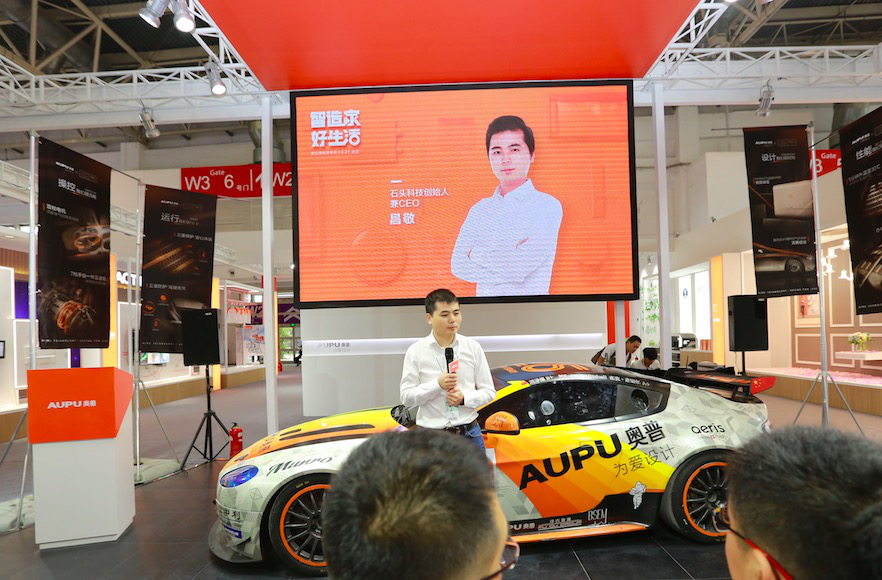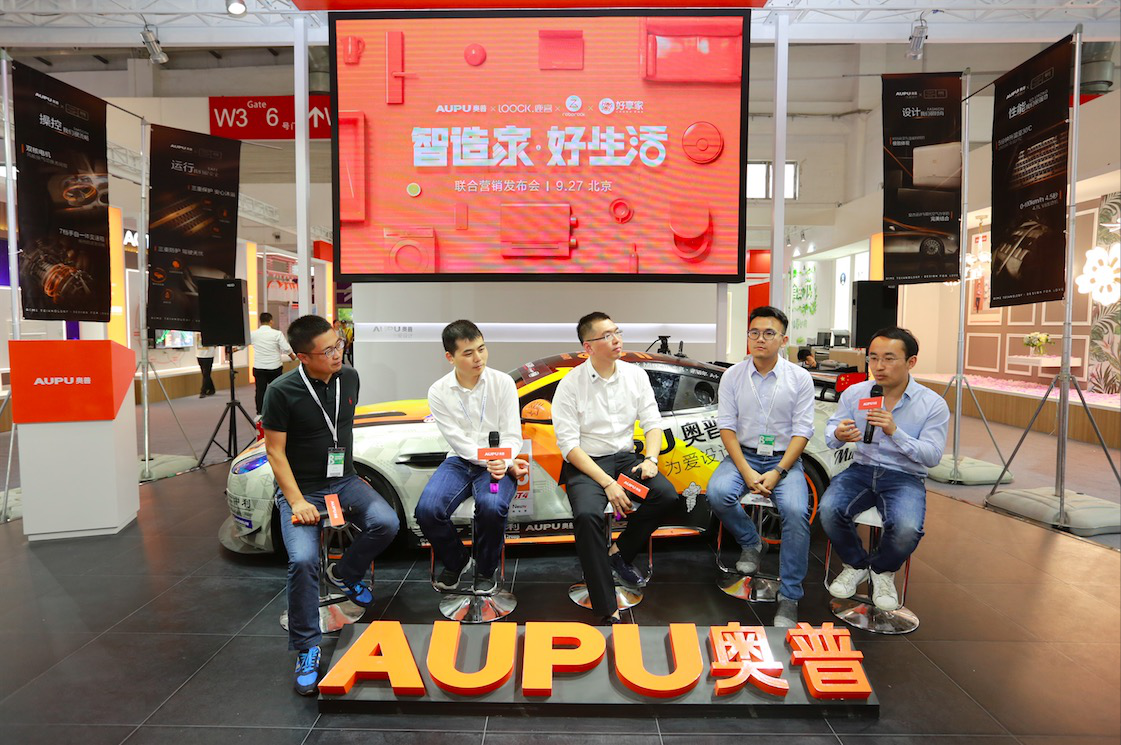|

# 奥普x云丁科技x石头科技x好享家 碰撞智能家居火花

随着“80、90”后主力年轻消费群体的迅速崛起，消费需求从满足基本日常生活转变为追求高品质舒适生活。对于居家产品，消费者更倾向于选择智能化、个性化、高品质、体验度更好的产品。

凭借在行业多年领跑的实力、对消费者需求的敏锐洞察力以及行业趋势的精准判断，奥普通过产品的不断创新，满足新时期的消费需求。

当奥普、云丁科技、石头科技、好享家四个品牌强强联合，足以给家带来一场智的改变，并持续为消费者提供品质生活。试想一下：你通过手机APP可以给亲友远程开门，也能收到陌生人靠近的报警。沐浴时，喊一声奥普管家就可以自由享受温暖和清新，而当走出浴室时，扫地机器人已经帮你做完了清洁工作。

以智会友，上演一场品质生活的体验。科技将使生活有多智能，还要看是否让人们的居住环境更健康、安全、舒适。

9月27日下午，奥普联合云丁科技、石头科技、好享家三大热门智能品牌发起以“智造家·好生活”为主题的联合营销发布会，在北京国际建材展奥普展位W3-B07举行。发布会前夕，居然之家董事长汪林朋先生亲临现场，并给予鼓励和支持。居然之家总裁王宁、石头科技创始人兼CEO昌敬、云丁科技创始人兼CEO陈彬、好享家联合创始人汪浩、腾讯家居全国总编辑张永志、奥普家居CEO吴兴杰出席本次发布会。

发布会伊始，居然之家总裁王宁先生作为展会东道主，表示很高兴行业内的佼佼者能够欢聚一堂，为大家分享使家居走向智能的产品，居然之家也会不遗余力的推动智能产品的发展，为家居智能化起到带头发展作用。随后奥普家居CEO吴兴杰向大家介绍道，智能最重要的是让生活更便捷，比如语音控制的奥普智能晾衣机，当拿着洗完的衣服准备晾时，不用手动降下晾衣机，省去复杂操作，节约有效时间。真正的智能，是有温度的，是让人们的家居生活更加地便捷美好。今天和另外三位大咖相聚于此，是为消费者打造更个性、高品质、更便捷的智能生活体验。比较有趣的是石头科技创始人兼CEO昌敬先生分享的小故事，石头S55扫地机器人解决了家庭矛盾，不用因为谁应该扫地纠结。扫地机器人最容易发生的情况就是漏扫和遇障碍物卡顿，石头可以全面覆盖不漏扫、APP预约划区清扫、灵敏适应各种环境。云丁科技创始人兼CEO陈彬的一句“指纹识别0.4秒开锁”引起了在座的兴趣，classic电磁防护设计有效保障家的安全，一把锁的使命就是守好家的第一道关卡。好享家联合创始人汪浩提到，好享家围绕顾客打造全方位舒适的集成体验，提升用户家庭所有设备的整体使用感，让真正的智能无处不在。智能产品最重要的点在于“通人性”，它最终解决的是体验感和效率的问题，在接下来的沙龙环节，腾讯家居全国总编辑张永志先生和四位大咖共同探讨了未来家居的发展。石头科技创始人兼CEO昌敬表示产品会朝着更全面的智能发展，未来希望打造的扫地机器人可以不单是清洁，还能做到整理，彻底释放双手，让生活更加轻松。

云丁科技创始人兼CEO陈彬表示，鹿客智能门锁会努力做到更安全、更便捷、更智能，比如联合锁业协会、公安部一起去制定出智能门锁更安全的标准，让产品可以有跟家人的温馨互动等，给用户带来更安全、智能和舒适的体验。

好享家联合创始人汪浩主要考虑给消费者更多的舒适体验，他提到，未来家庭端，所有设备都会智能化，而随着行业的进步与发展，厂商端和前端营销企业会达成共识，共同生产消费者心仪的产品。

奥普家居CEO 吴兴杰表示，有温度的智能，不单是为了智能而智能，未来的智能是一定是开放的，是由各个专业的集合，万物互联才能给消费者带来便捷，让消费者在产品功能的体验上感受到温度。随着科技的发展，从智能门锁到智能家居，再到其他的行业，人工智能逐渐成为推动众多传统行业进一步发展的重要驱动力，提升以技术为核心的产品力。除了加强研发之外，就是让技术更好地为人们和家庭服务。

奥普、云丁科技、石头科技、好享家四个行业大咖品牌，站在智能家居前沿的企业，因开放和包容的心态走到一起，共同发声，联合推动智能家居的发展，同时也期待更多品牌能够加入到智能大军，一起打造智能化、个性化、高品质、高体验度的智能生活新体验。

`声明：本文由入驻焦点开放平台的作者撰写，除焦点官方账号外，观点仅代表作者本人，不代表焦点立场错误信息举报电话： 400-099-0099，邮箱：jubao@vip.sohu.com，或点此进行意见反馈，或点此进行举报投诉。`A B C D E F G H J K L M N P Q R S T W X Y Z
A - B - C - D - E
• A
• 鞍山
• 安庆
• 安阳
• 安顺
• 安康
• 澳门
• B
• 北京
• 保定
• 包头
• 巴彦淖尔
• 本溪
• 蚌埠
• 亳州
• 滨州
• 北海
• 百色
• 巴中
• 毕节
• 保山
• 宝鸡
• 白银
• 巴州
• C
• 承德
• 沧州
• 长治
• 赤峰
• 朝阳
• 长春
• 常州
• 滁州
• 池州
• 长沙
• 常德
• 郴州
• 潮州
• 崇左
• 重庆
• 成都
• 楚雄
• 昌都
• 慈溪
• 常熟
• D
• 大同
• 大连
• 丹东
• 大庆
• 东营
• 德州
• 东莞
• 德阳
• 达州
• 大理
• 德宏
• 定西
• 儋州
• 东平
• E
• 鄂尔多斯
• 鄂州
• 恩施
F - G - H - I - J
• F
• 抚顺
• 阜新
• 阜阳
• 福州
• 抚州
• 佛山
• 防城港
• G
• 赣州
• 广州
• 桂林
• 贵港
• 广元
• 广安
• 贵阳
• 固原
• H
• 邯郸
• 衡水
• 呼和浩特
• 呼伦贝尔
• 葫芦岛
• 哈尔滨
• 黑河
• 淮安
• 杭州
• 湖州
• 合肥
• 淮南
• 淮北
• 黄山
• 菏泽
• 鹤壁
• 黄石
• 黄冈
• 衡阳
• 怀化
• 惠州
• 河源
• 贺州
• 河池
• 海口
• 红河
• 汉中
• 海东
• 怀来
• I
• J
• 晋中
• 锦州
• 吉林
• 鸡西
• 佳木斯
• 嘉兴
• 金华
• 景德镇
• 九江
• 吉安
• 济南
• 济宁
• 焦作
• 荆门
• 荆州
• 江门
• 揭阳
• 金昌
• 酒泉
• 嘉峪关
K - L - M - N - P
• K
• 开封
• 昆明
• 昆山
• L
• 廊坊
• 临汾
• 辽阳
• 连云港
• 丽水
• 六安
• 龙岩
• 莱芜
• 临沂
• 聊城
• 洛阳
• 漯河
• 娄底
• 柳州
• 来宾
• 泸州
• 乐山
• 六盘水
• 丽江
• 临沧
• 拉萨
• 林芝
• 兰州
• 陇南
• M
• 牡丹江
• 马鞍山
• 茂名
• 梅州
• 绵阳
• 眉山
• N
• 南京
• 南通
• 宁波
• 南平
• 宁德
• 南昌
• 南阳
• 南宁
• 内江
• 南充
• P
• 盘锦
• 莆田
• 平顶山
• 濮阳
• 攀枝花
• 普洱
• 平凉
Q - R - S - T - W
• Q
• 秦皇岛
• 齐齐哈尔
• 衢州
• 泉州
• 青岛
• 清远
• 钦州
• 黔南
• 曲靖
• 庆阳
• R
• 日照
• 日喀则
• S
• 石家庄
• 沈阳
• 双鸭山
• 绥化
• 上海
• 苏州
• 宿迁
• 绍兴
• 宿州
• 三明
• 上饶
• 三门峡
• 商丘
• 十堰
• 随州
• 邵阳
• 韶关
• 深圳
• 汕头
• 汕尾
• 三亚
• 三沙
• 遂宁
• 山南
• 商洛
• 石嘴山
• T
• 天津
• 唐山
• 太原
• 通辽
• 铁岭
• 泰州
• 台州
• 铜陵
• 泰安
• 铜仁
• 铜川
• 天水
• 天门
• W
• 乌海
• 乌兰察布
• 无锡
• 温州
• 芜湖
• 潍坊
• 威海
• 武汉
• 梧州
• 渭南
• 武威
• 吴忠
• 乌鲁木齐
X - Y - Z
• X
• 邢台
• 徐州
• 宣城
• 厦门
• 新乡
• 许昌
• 信阳
• 襄阳
• 孝感
• 咸宁
• 湘潭
• 湘西
• 西双版纳
• 西安
• 咸阳
• 西宁
• 仙桃
• 西昌
• Y
• 运城
• 营口
• 盐城
• 扬州
• 鹰潭
• 宜春
• 烟台
• 宜昌
• 岳阳
• 益阳
• 永州
• 阳江
• 云浮
• 玉林
• 宜宾
• 雅安
• 玉溪
• 延安
• 榆林
• 银川
• Z
• 张家口
• 镇江
• 舟山
• 漳州
• 淄博
• 枣庄
• 郑州
• 周口
• 驻马店
• 株洲
• 张家界
• 珠海
• 湛江
• 肇庆
• 中山
• 自贡
• 资阳
• 遵义
• 昭通
• 张掖
• 中卫

1室1厅1厨1卫1阳台

1
2
3
4
5

0
1
2

1

1

0
1
2
3报名成功，资料已提交审核A B C D E F G H J K L M N P Q R S T W X Y Z
A - B - C - D - E
• A
• 鞍山
• 安庆
• 安阳
• 安顺
• 安康
• 澳门
• B
• 北京
• 保定
• 包头
• 巴彦淖尔
• 本溪
• 蚌埠
• 亳州
• 滨州
• 北海
• 百色
• 巴中
• 毕节
• 保山
• 宝鸡
• 白银
• 巴州
• C
• 承德
• 沧州
• 长治
• 赤峰
• 朝阳
• 长春
• 常州
• 滁州
• 池州
• 长沙
• 常德
• 郴州
• 潮州
• 崇左
• 重庆
• 成都
• 楚雄
• 昌都
• 慈溪
• 常熟
• D
• 大同
• 大连
• 丹东
• 大庆
• 东营
• 德州
• 东莞
• 德阳
• 达州
• 大理
• 德宏
• 定西
• 儋州
• 东平
• E
• 鄂尔多斯
• 鄂州
• 恩施
F - G - H - I - J
• F
• 抚顺
• 阜新
• 阜阳
• 福州
• 抚州
• 佛山
• 防城港
• G
• 赣州
• 广州
• 桂林
• 贵港
• 广元
• 广安
• 贵阳
• 固原
• H
• 邯郸
• 衡水
• 呼和浩特
• 呼伦贝尔
• 葫芦岛
• 哈尔滨
• 黑河
• 淮安
• 杭州
• 湖州
• 合肥
• 淮南
• 淮北
• 黄山
• 菏泽
• 鹤壁
• 黄石
• 黄冈
• 衡阳
• 怀化
• 惠州
• 河源
• 贺州
• 河池
• 海口
• 红河
• 汉中
• 海东
• 怀来
• I
• J
• 晋中
• 锦州
• 吉林
• 鸡西
• 佳木斯
• 嘉兴
• 金华
• 景德镇
• 九江
• 吉安
• 济南
• 济宁
• 焦作
• 荆门
• 荆州
• 江门
• 揭阳
• 金昌
• 酒泉
• 嘉峪关
K - L - M - N - P
• K
• 开封
• 昆明
• 昆山
• L
• 廊坊
• 临汾
• 辽阳
• 连云港
• 丽水
• 六安
• 龙岩
• 莱芜
• 临沂
• 聊城
• 洛阳
• 漯河
• 娄底
• 柳州
• 来宾
• 泸州
• 乐山
• 六盘水
• 丽江
• 临沧
• 拉萨
• 林芝
• 兰州
• 陇南
• M
• 牡丹江
• 马鞍山
• 茂名
• 梅州
• 绵阳
• 眉山
• N
• 南京
• 南通
• 宁波
• 南平
• 宁德
• 南昌
• 南阳
• 南宁
• 内江
• 南充
• P
• 盘锦
• 莆田
• 平顶山
• 濮阳
• 攀枝花
• 普洱
• 平凉
Q - R - S - T - W
• Q
• 秦皇岛
• 齐齐哈尔
• 衢州
• 泉州
• 青岛
• 清远
• 钦州
• 黔南
• 曲靖
• 庆阳
• R
• 日照
• 日喀则
• S
• 石家庄
• 沈阳
• 双鸭山
• 绥化
• 上海
• 苏州
• 宿迁
• 绍兴
• 宿州
• 三明
• 上饶
• 三门峡
• 商丘
• 十堰
• 随州
• 邵阳
• 韶关
• 深圳
• 汕头
• 汕尾
• 三亚
• 三沙
• 遂宁
• 山南
• 商洛
• 石嘴山
• T
• 天津
• 唐山
• 太原
• 通辽
• 铁岭
• 泰州
• 台州
• 铜陵
• 泰安
• 铜仁
• 铜川
• 天水
• 天门
• W
• 乌海
• 乌兰察布
• 无锡
• 温州
• 芜湖
• 潍坊
• 威海
• 武汉
• 梧州
• 渭南
• 武威
• 吴忠
• 乌鲁木齐
X - Y - Z
• X
• 邢台
• 徐州
• 宣城
• 厦门
• 新乡
• 许昌
• 信阳
• 襄阳
• 孝感
• 咸宁
• 湘潭
• 湘西
• 西双版纳
• 西安
• 咸阳
• 西宁
• 仙桃
• 西昌
• Y
• 运城
• 营口
• 盐城
• 扬州
• 鹰潭
• 宜春
• 烟台
• 宜昌
• 岳阳
• 益阳
• 永州
• 阳江
• 云浮
• 玉林
• 宜宾
• 雅安
• 玉溪
• 延安
• 榆林
• 银川
• Z
• 张家口
• 镇江
• 舟山
• 漳州
• 淄博
• 枣庄
• 郑州
• 周口
• 驻马店
• 株洲
• 张家界
• 珠海
• 湛江
• 肇庆
• 中山
• 自贡
• 资阳
• 遵义
• 昭通
• 张掖
• 中卫• 手机• 分享
• 设计
免费设计
• 计算器
装修计算器
• 入驻
合作入驻
• 联系
联系我们
• 置顶
返回顶部